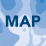# Hello everybody I need some help about python scripting to create a square buffer around points feature class ?

8390
9
01-12-2015 06:21 PMNew Contributor II

If there is a problem by giving me the script , I just need some explanation about how can I start scripting the square buffer?

I'm a beginner python programmer, I'm looking forward to be professional in python , and I hope that any one will help me !

1 Solution

Accepted SolutionsbyEsri Esteemed Contributor

... and if you want to create an output that contains the attributes of the input, you can try this:

```import arcpy, os

def main():
arcpy.env.overwriteOutput = True

# inputs
fc_in = r'D:\Xander\EPM\Datos pruebas\gdb\datos erase.gdb\points03'
width = 0.005 # with of the output rectangle
height = 0.005 # height of the output rectangle

# output
fc_out = r'D:\Xander\EPM\Datos pruebas\gdb\datos erase.gdb\rectangles3'

# create empty output featureclass
sr = arcpy.Describe(fc_in).spatialReference
out_ws, out_name = os.path.split(fc_out)
arcpy.CreateFeatureclass_management(out_ws, out_name, "POLYGON", fc_in, "DISABLED", "DISABLED", sr)

# make fields list
flds_use = correctFieldList(arcpy.ListFields(fc_in))

# create insert cursor
with arcpy.da.InsertCursor(fc_out, flds_use) as curs_out:

# loop through input features
with arcpy.da.SearchCursor(fc_in, flds_use) as curs_in:
for row_in in curs_in:
polygon = createRectangleFromPoint(row_in.firstPoint, width, height, sr)
row_out = buildRow(row_in, flds_use, polygon)
curs_out.insertRow(row_out)

def createRectangleFromPoint(pnt, width, height, sr):
arrPnts = arcpy.Array()
pnt2 = arcpy.Point(pnt.X - width, pnt.Y - height)
pnt2 = arcpy.Point(pnt.X - width, pnt.Y + height)
pnt2 = arcpy.Point(pnt.X + width, pnt.Y + height)
pnt2 = arcpy.Point(pnt.X + width, pnt.Y - height)
pnt2 = arcpy.Point(pnt.X - width, pnt.Y - height)
return arcpy.Polygon(arrPnts, sr)

def correctFieldList(flds):
flds_use = ['Shape@']
fldtypes_not = ['Geometry', 'Guid', 'OID']
for fld in flds:
if not fld.type in fldtypes_not:
flds_use.append(fld.name)
return flds_use

def buildRow(row_in, flds_use, polygon):
try:
lst_in = list(row_in)
lst_in = polygon
return tuple(lst_in)
except Exception, e:
print("buildRow exception: {0}".format(e))
return None

if __name__ == '__main__':
main()```
9 RepliesbyMVP Legendary Contributor

See Xander Bakker's diamond buffer script...a little bit of fiddling and you can turn a diamond into a squareOccasional Contributor

Hi,

Thanks and Regards,

VenkatbyEsri Esteemed Contributor

Below the code based on the thread mentioned by Dan Patterson‌ adapted to create squares or rectangles. Change lines 6 and 11 to point to your input and output (will be created) and change the sizes on lines 7 and 8. For some more snippets with Python have a look at: https://community.esri.com/docs/DOC-1927

```import arcpy
def main():
arcpy.env.overwriteOutput = True

# inputs
fc = r'D:\Xander\Rectangles\data.gdb\points'
width = 0.005 # with of the output rectangle
height = 0.005 # height of the output rectangle

# output
fcout = r'D:\Xander\Rectangles\data.gdb\rectangles'

sr = arcpy.Describe(fc).spatialReference
features = []
with arcpy.da.SearchCursor(fc, ("SHAPE@")) as cursor:
for row in cursor:
rectangle = createRectangleFromPoint(row.firstPoint, width, height, sr)
features.append(rectangle)

# write to output
arcpy.CopyFeatures_management(features, fcout)

def createRectangleFromPoint(pnt, width, height, sr):
arrPnts = arcpy.Array()
pnt2 = arcpy.Point(pnt.X - width, pnt.Y - height)
pnt2 = arcpy.Point(pnt.X - width, pnt.Y + height)
pnt2 = arcpy.Point(pnt.X + width, pnt.Y + height)
pnt2 = arcpy.Point(pnt.X + width, pnt.Y - height)
pnt2 = arcpy.Point(pnt.X - width, pnt.Y - height)
return arcpy.Polygon(arrPnts, sr)

if __name__ == '__main__':
main()```byEsri Esteemed Contributor

... and if you want to create an output that contains the attributes of the input, you can try this:

```import arcpy, os

def main():
arcpy.env.overwriteOutput = True

# inputs
fc_in = r'D:\Xander\EPM\Datos pruebas\gdb\datos erase.gdb\points03'
width = 0.005 # with of the output rectangle
height = 0.005 # height of the output rectangle

# output
fc_out = r'D:\Xander\EPM\Datos pruebas\gdb\datos erase.gdb\rectangles3'

# create empty output featureclass
sr = arcpy.Describe(fc_in).spatialReference
out_ws, out_name = os.path.split(fc_out)
arcpy.CreateFeatureclass_management(out_ws, out_name, "POLYGON", fc_in, "DISABLED", "DISABLED", sr)

# make fields list
flds_use = correctFieldList(arcpy.ListFields(fc_in))

# create insert cursor
with arcpy.da.InsertCursor(fc_out, flds_use) as curs_out:

# loop through input features
with arcpy.da.SearchCursor(fc_in, flds_use) as curs_in:
for row_in in curs_in:
polygon = createRectangleFromPoint(row_in.firstPoint, width, height, sr)
row_out = buildRow(row_in, flds_use, polygon)
curs_out.insertRow(row_out)

def createRectangleFromPoint(pnt, width, height, sr):
arrPnts = arcpy.Array()
pnt2 = arcpy.Point(pnt.X - width, pnt.Y - height)
pnt2 = arcpy.Point(pnt.X - width, pnt.Y + height)
pnt2 = arcpy.Point(pnt.X + width, pnt.Y + height)
pnt2 = arcpy.Point(pnt.X + width, pnt.Y - height)
pnt2 = arcpy.Point(pnt.X - width, pnt.Y - height)
return arcpy.Polygon(arrPnts, sr)

def correctFieldList(flds):
flds_use = ['Shape@']
fldtypes_not = ['Geometry', 'Guid', 'OID']
for fld in flds:
if not fld.type in fldtypes_not:
flds_use.append(fld.name)
return flds_use

def buildRow(row_in, flds_use, polygon):
try:
lst_in = list(row_in)
lst_in = polygon
return tuple(lst_in)
except Exception, e:
print("buildRow exception: {0}".format(e))
return None

if __name__ == '__main__':
main()```by
Occasional Contributor II

Is it possible to use this code using a width and height attribute field in the input file for different widths and heights? Thank you.

I tried the following but get an error: pnt2 = arcpy.Point(pnt.X - width, pnt.Y - height)
TypeError: unsupported operand type(s) for -: 'float' and 'str'

width = "float(!newWidth!)"

height = "float(!newHeight!)"byEsri Esteemed Contributor

You cannot define the width like you did and as you can notice in the error you cannot mix numeric and text values. You will probably have to do something like this:

``````    fld_width = "name of your width field"
fld_height = "name of your height field"

# ...

with arcpy.da.SearchCursor(fc_in, (flds_use, fld_width, fld_height)) as curs_in:
for row_in in curs_in:
width = row_in
height = row_in
polygon = createRectangleFromPoint(row_in.firstPoint, width, height, sr)
‍‍‍‍‍‍‍‍‍‍``````

In the beginning you will have to define the names of your width and height field and in the search cursor you will have to include the field names in the list of fields. Inside the loop you will have to read the values and use them in the CreateRectangleFromPoint function.by
Occasional Contributor II

Thanks for your help! I used "width" twice because I am just creating squares.by
Occasional Contributor II

After running this code I believe the "width" and "height" values are actually half of the width and half of the height of the output rectangle. In other words width and height are radii values.byEsri Esteemed Contributor

Hi louchios ,

That is a correct observation although it would be better to avoid confusion to maintain your width and height values and correct the code to use width/2 and height/2 in creating the corner points.